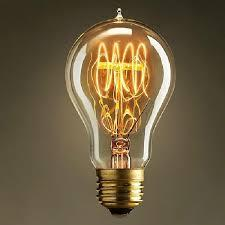# UN 1

If we add to an unknown number his quarter, we get 210. Identify unknown number.

x =  168

### Step-by-step explanation:

x+x/4=210

5x = 840

x = 168

Our simple equation calculator calculates it.Did you find an error or inaccuracy? Feel free to write us. Thank you!Tips to related online calculators
Need help to calculate sum, simplify or multiply fractions? Try our fraction calculator.
Do you have a linear equation or system of equations and looking for its solution? Or do you have a quadratic equation?

## Related math problems and questions:

• Unknown xIf we add to unknown number 21, then divide by 6 and then subtract 51, we get back an unknown number. What is this unknown number?
• Unknown numberIf I reduced the sum of the numbers 70 and the unknown number three times, I would get 100. what is the unknown number?
• Unknown numberFind the unknown number equal to a quarter of a fifth of a number, which is by 152 more than an unknown number.
• NumberI think the number. If I add to its third seven I get same as when to its quarter add 8. Which is the number?
• Unknown numberFind an unknown number of which 1/5 is 40 greater than one-tenth of that number.
• Two numbersThe sum of two numbers is 1. Identify this two numbers if you know that the half of first is equal to the third of second number.
• Unknown number 24f we add 20, we get 50% of its triple. What is this unknown number?
• Unknown number 5I think of an unknown number. If we enlarge it five times then subtract 3 and the result decreases by 75% we get one greater than the number. What number am I thinking of?
• If weIf we increase the unknown number by 4%, we get 780. Determine the unknown number.
• Ratio of two unknown numbersTwo numbers are given. Their sum is 30. We calculate one-sixth of a larger number and add to both numbers. So we get new numbers whose ratio is 5:7. Which two numbers were given?
• Number with onesThe first digit of the number is 1, if we move this digit to the end we get a 3 times higher number, which is the number?
• Percentage - fractionsAbout what percentage we must increase number 1/6 to get number 1/3?
• Unknown numberSamuel wrote unknown number. Then he had add 200000 to the number and the result multiply by three. When it calculated he was surprised, because the result would have received anyway, if write digit to the end of original number. Find unknown number.
• I think numberI think number.When I add 841 to it and subtract 157, I get a number that is 22 greater than 996. What number I thinking?
• Unknown number 716% of the unknown number is by 21 less than unknown number itself. Determine the natural unknown number.
• One-thirdA one-third of unknown number is equal to five times as great as the difference of the same unknown number and number 28. Determine the unknown number.
• Unknown numberI think the number. I'll reduce it to its one-third. The result is then increased by one-third, and I get the number 12.